### 15.4.3.2 Lie algebra of the system distribution

Now suppose that a set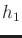,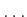,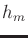of vector fields is given as a driftless control-affine system, as in (15.53). Its associated distribution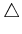is interpreted as a vector space with coefficients in, and the Lie bracket operation was given by (15.81). It can be verified that the Lie bracket operation in (15.81) satisfies the required axioms for a Lie algebra.

As observed in Examples 15.9 and 15.10, the Lie bracket may produce vector fields outside of. By defining the Lie algebra ofto be all vector fields that can be obtained by applying Lie bracket operations, a potentially larger distribution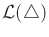is obtained. The Lie algebra can be expressed using the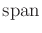notation by including,,and all independent vector fields generated by Lie brackets. Note that no more thanindependent vector fields can possibly be produced.

Example 15..16 (The Lie Algebra of the Differential Drive)   The Lie algebra of the differential drive (15.54) is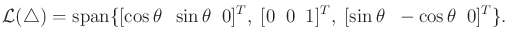(15.101)

This uses the Lie bracket that was computed in (15.82) to obtain a three-dimensional Lie algebra. No further Lie brackets are needed because the maximum number of independent vector fields has been already obtained.Example 15..17 (A Lie Algebra That Involves Nested Brackets)   The previous example was not very interesting because the Lie algebra was generated after computing only one bracket. Suppose that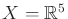and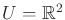. In this case, there is room to obtain up to three additional, linearly independent vector fields. The dimension of the Lie algebra may be any integer from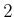to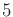.

Let the system be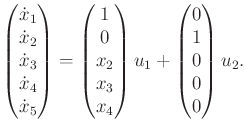(15.102)

This is a chained-form system, which is a concept that becomes important in Section 15.5.2.

The first Lie bracket produces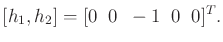(15.103)

Other vector fields that can be obtained by Lie brackets are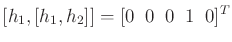(15.104)

and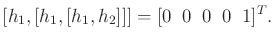(15.105)

The resulting five vector fields are independent over all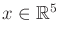. This includes,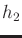, and the three obtained from Lie bracket operations. Independence can be established by placing them into a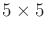matrix,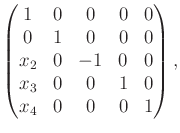(15.106)

which has full rank for all. No additional vector fields can possibly be independent. Therefore, the five-dimensional Lie algebra is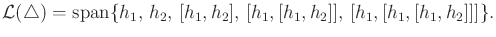(15.107)Steven M LaValle 2020-08-14